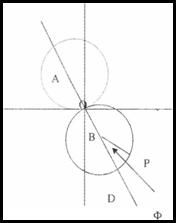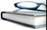Source: Textile Review

Theoritical Concept of DRF Yarn ProductionIn the figure a three dimensional Cartesian representing the yarn axis lies along OZ (Perpendicular to the plane of the paper). The rotating vector OB advancing
generates the helix representing the strand axis at a constant rate along the two-fold yarn axis

The point P is the position of a fiber on the surface of the strand so that P is defined by the angle

PBD = Ф

Treloat shows that the total torsion of each strand (sp) as it lies in the two- fold yarns consists of the some of the torsion relative to the principal plane of curvature of the strand axis and the rate of rotation of the plane of curvature of the strand axis and the rate of rotation of the plane of curvature and that the total torsion